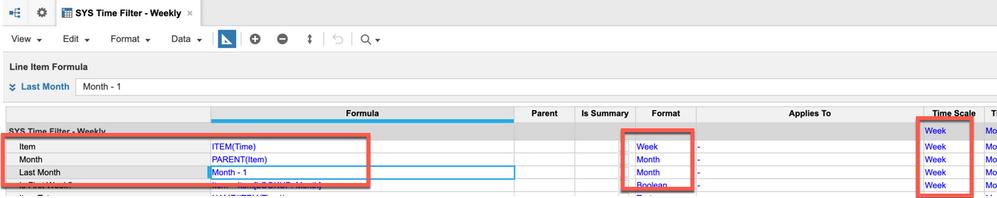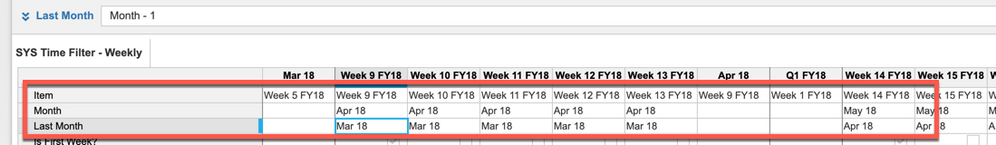# Week Calculation-Alternate way to put number of weeks in a yearHi Everyone

I am using '52' to show previous year data of same month by subtracting: 'PY Month(week)' - 52

Is there a function or alternate way to use replace '52' and not use it in formula as a number but a derived value from some function or calculation?

Thanks!

Tagged:

•• Week
• Month
• Last MonthRob

•Assuming that the target module is dimensioned by time periods you can use the OFFSET function. This allows you to offset the reference by a stated number of time periods. If your using weeks then you will use 52, months you would use 12 and so on.

Therefore, in the you example use; OFFSET(DATA,-52) the negative value indicates that the function is going backs to retrieve the required value.

The offset value can be referenced to an input module where as a user you can flex the value but if its always going to be 52 weeks prior then hard coding it here is ok!

Note that this function only works if the data and target have the same time dimension.

•Hi @Aniruddha ,

Can you clarify your question? Are you looking to dynamically calculate # of weeks in a given year? 52 for normal years + 53 for other fiscal years?

Trying to understand your use case and possibly propose some solutions based on that.